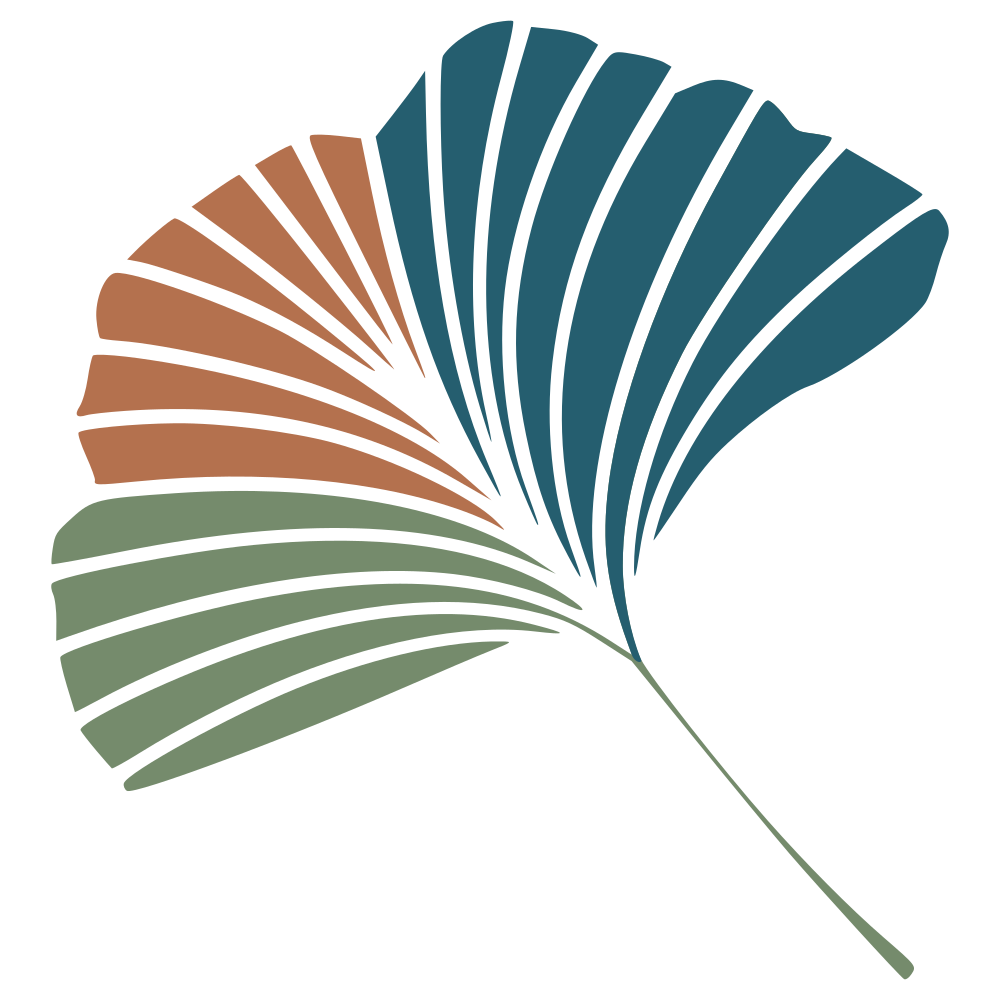# Graduate classes in biological modeling are hard

(because undergraduate classes in modeling are easy)

In a few days, I will start the new iteration of my Modeling and Computational Biology class, which is a fancy way of saying that we will think about the representation of biological situations into the language of mathematics, and then about the implementation of these representations in the language of computers. It is a graduate class, where I rely a lot on “live mathematics”. It is, also, somewhat difficult, because there is a lot of overhead associated with learning the material.

In an undergraduate level class on population dynamics, where students start seeing things like $\dot n = rn(1-n/K)$, we usually do not go into the depth of the models - they just are. I should now give an important caveat; I only taught undergraduate population biology once, and I never took it, because I am a fake ecologist. But models are mostly presented as canonical equations, you can look them up in a table, they have names, and there’s like 6 of them.

My ambition for a graduate class in modeling is to make the process of modeling, well, a process. Something that starts by taking a biological situation, expressing it in equations, conducting a reasonably formal analysis of it, and then performing simulations. The steep change in difficulty when addressing the problem this way is that the amount of mathematical bagage required is much larger. Even the best textbook for this use-case (Otto & Day) assumes a fair bit of mathematical knowledge. As an example, last year, we only reached a point where “what is a differential equation?” stopped being an issue after three weeks (and a few re-watchs of 3blue1browns’s The Essence of Calculus series).

Most analyses of dynamical systems require to draw on concepts of both calculus and linear algebra. Even when taking a shortcut during local stability analysis by using the Routh-Hurwitz criteria, students need an understanding of the Jacobian matrix, its trace, and its determinant. Sometimes an intuition of what these values are can help, but often the closest intuition is an analogy to another mathematical concept. It’s just hard.

Part of the difficulty in building these skills, to put it in terms Mr. and Mrs. Tweedy would use, is that undergraduate training focuses on eating the pie thats comes out, but graduate training is supposed to be all about where to put the chicken and how the machine works. And as much as in both instances we are manipulating equations, what we are doing with them is wholly different in the two situations. In short, graduate modeling classes are not a quantitative change, but a qualitative one.

The other part of the difficulty is time. To put it gently, the quantitative education of most biologists at the undergraduate level is terrible. Lackluster. Non-existant. Curriculums that have not been updated in a while lead to low computational literacy, low mathematical literacy, and you can trace a straight line from this to how hard learning biological modeling is on students. I have stopped counting the number of times the question of “decreasing the standards for graduate classes” versus “increasing the standard for undergraduate classes” popped up in my life, but it would be correct, at this point, to classify it as a periodic event. And because the undergraduate curriculum is lacking in quantitative content in most places, there is a lot of catching up to do.

In truth, graduate classes do not have to be that intense. It would take fairly minor modifications to the undergraduate curriculum to make them a whole lot easier. Give students a more robust foundation in the very basics of mathematics biologists use (most of it is high-school level!); give students an actual foundation in programming (things are true or false, write functions, loops are a thing). Were this amount of knowledge communicated over a few year, it would make moving into more advanced topics easier.

And to drive this point across a little further - these skills are not useful only to the handful of students (if that) who want to get involved in modeling as part of their scientific identity; they are required to understand the theoretical literature, and to account for theoretical results even in very deeply empirical applications. Because most biologists will not end up doing modeling; but an exhaustive overview of the field demands that students be able to understand what models communicate, because theory contributes to the development of ideas as much as empirical approaches do. And because modeling is such a tricky practice, a surface understanding is not enough.